## Special symbols

The LaTeX language has a wide variety of special symbols for which markup commands have already been defined. These range from accents and greek letters to exotic mathematical operators.

These LaTeX's symbols are grouped together more or less according to function. Some of these symbols are primarily for use in text; most of them are mathematical symbols and can only be used in LaTeX's math mode. Most tables are excerpted from the LaTeX Command Summary (Botway & Biemesderfer 1989, Providence, RI: TeX Users Group) and reproduced here courtesy of the AAS.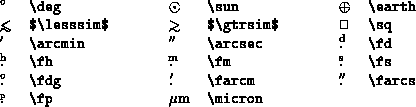Table 1: Special symbols for NOAOprop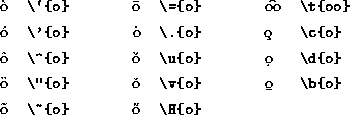Table 2: Text-mode accents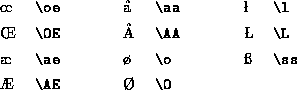Table 3: National symbols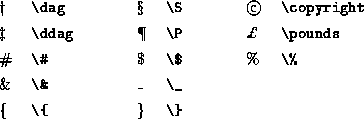Table 4: Miscellaneous symbols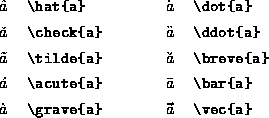Table 5: Math-mode accentsTable 6: Greek letters (math mode)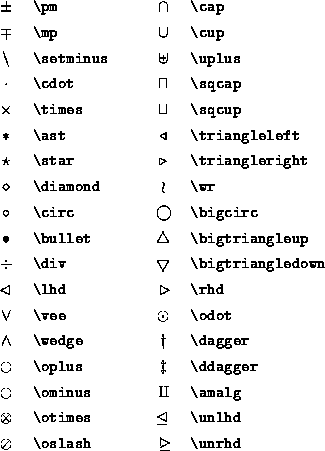Table 7: Binary operations (math mode)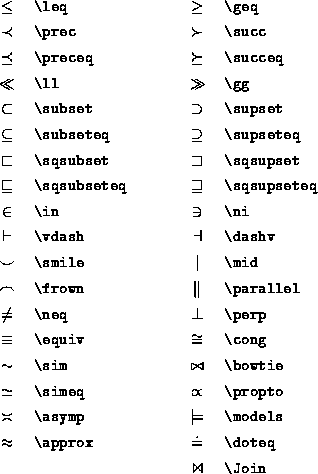Table 8: Relations (math mode)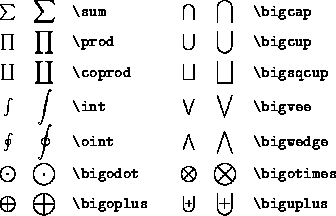Table 9: Variable-sized symbols (math mode)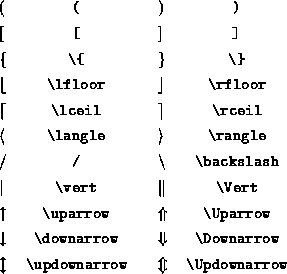Table 10: Delimiters (math mode)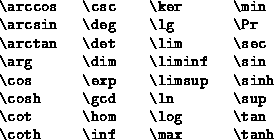Table 11: Function names (math mode)Table 12: Arrows (math mode)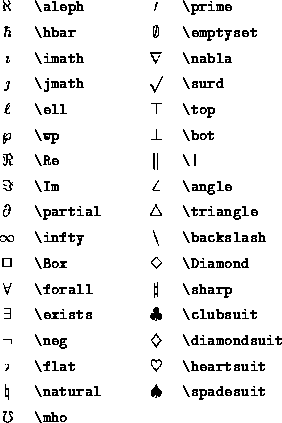Table 13: Miscellaneous symbols (math mode)